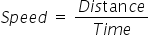Mathematics
Easy

Question

# An aeroplane flies with a constant speed of 600 miles per 5 hours. If it travels at the same rate, distance travelled in 8 hours by the plane is ________________.

## 960900850750Hint:

## The correct answer is: 960

### The given values of distance and time are as follows:Distance = 600 milesTime = 5 hoursWe have to find the speed as it is given that the plane is flying at constant speed.Now, as the rate or speed is constant, we can use this value to find the value of the distance travelled for at any other time.We have to find the distance travelled in 8 hours.Let’s see the formulathe formula to find distance. We will multiply both sides by time.Distance = Speed × Time= 120 miles/ hour × 8 hours= 960 milesTherefore, the distance travelled is 960 miles.So the option which is ‘960’, is the right option.

We have to be careful about the units. Same quantities have same value.

### Related Questions to study#### With Turito Foundation.#### Get an Expert Advice From Turito.